# 1379. Find the same node in the cloned binary tree (Java / C + + / Python)

2022-01-31 17:42:08

This is my participation 11 The fourth of the yuegengwen challenge 9 God , Check out the activity details ：2021 One last more challenge

welcome 【 give the thumbs-up 】【 Collection 】【 Comment on 】~
It's not hard to give up , But persistence must be cool ~
I hope all of us can make a little progress every day ~
This paper is written by The white hat of the second leader ：https://juejin.cn/user/2771185768884824/posts Original blog ~

# 1379. Find the same node in the clone binary tree ：

Here are two binary trees , Primitive tree `original` And clone trees `cloned`, And one in the original tree `original` Target node in `target`.

among , Clone tree `cloned` It's a primitive tree `original` One of the copy .

Please find the tree `cloned` in , And `target` identical The node of , And return a reference to the node （ stay C/C++ Return... In a language with a pointer Node pointer , Other languages return the node itself ）.

# Be careful ：

1. you You can't For two binary trees , as well as `target` Make changes to the node .
2. Can only Return to the clone tree `cloned` References to existing nodes in .

Advanced ： If nodes with the same value are allowed in the tree , How will you answer ？

# Examples 1：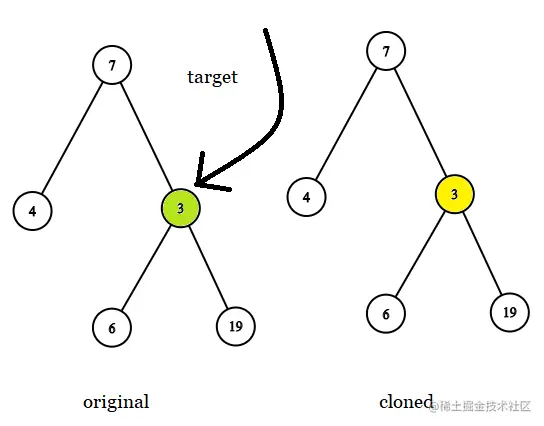`````` Input :
tree = [7,4,3,null,null,6,19], target = 3

Output :
3

explain :
The picture above shows the tree  original  and  cloned.target  Nodes in the tree  original  in , Mark it in green . The answer is trees  cloned  Yellow nodes in （ Other examples are similar ）.
Copy code ``````

# Examples 2：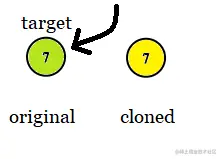`````` Input :
tree = , target =  7

Output :
7
Copy code ``````

# Examples 3：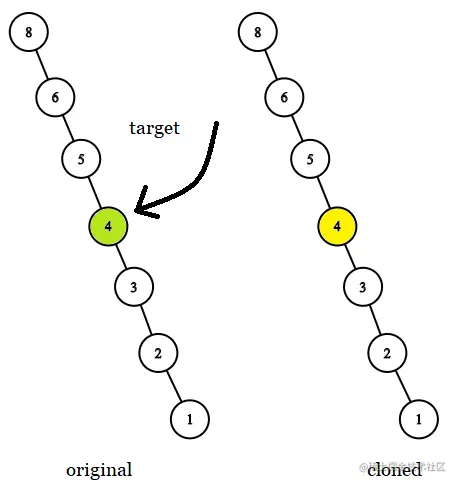`````` Input :
tree = [8,null,6,null,5,null,4,null,3,null,2,null,1], target = 4

Output :
4
Copy code ``````

# Examples 4：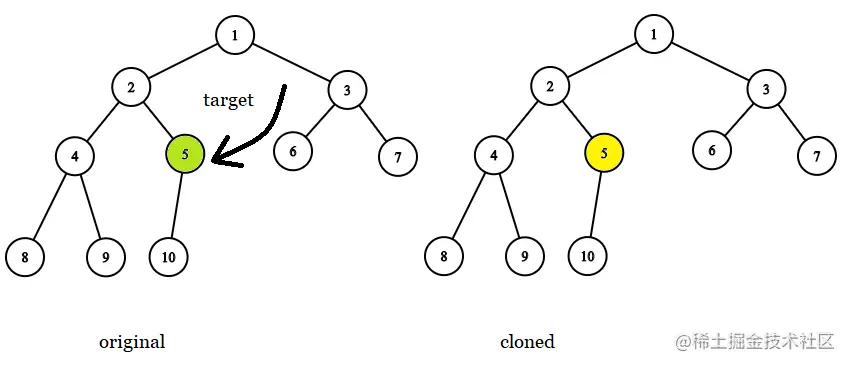`````` Input :
tree = [1,2,3,4,5,6,7,8,9,10], target = 5

Output :
5
Copy code ``````

# Examples 5：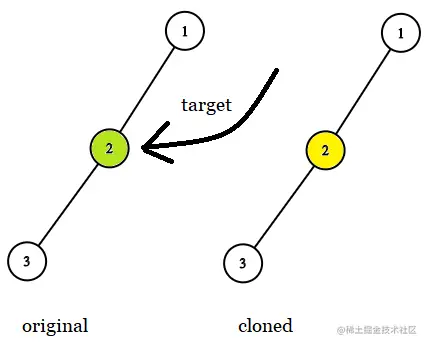`````` Input :
tree = [1,2,null,3], target = 2

Output :
2
Copy code ``````

# Tips ：

• The number of nodes in the tree ranges from [1, 104] .
• In the same tree , There are no nodes with the same value .
• `target` Nodes are trees `original` One of the nodes in , And it won't be `null` .

# analysis

• This algorithm problem needs a simple translation , Three parameters ： The first parameter `original` It's the original tree ; The second parameter `cloned` Is a clone copy of the first parameter ; The third parameter `target` Is the node we want to find , It is the first parameter `original` One of the nodes in , You need to find and return the second parameter `cloned` The corresponding node in .
• The hint says that in the same tree , There are no nodes with the same value . Therefore, some small partners may feel that the first parameter is very redundant , The second leader thinks so . We directly iterate over the second parameter `cloned` , Until you find and the third parameter `target` Just return a node with the same value .
• In fact, the first parameter is Advanced It's useful in the challenge , If nodes with the same value are allowed in the tree , Then you can't judge the same node by value .
• In this case, you need to use the first parameter `original` , Because the third parameter `target` Is a node in the original tree , So we can directly judge whether it is the same node according to the address .
• The second parameter `cloned` Is a clone copy of the first parameter , So they have the same structure , We just traverse the original tree and clone tree in the same order , You can find the answer .

## java

Non recursive traversal

``````/** * Definition for a binary tree node. * public class TreeNode { * int val; * TreeNode left; * TreeNode right; * TreeNode(int x) { val = x; } * } */

class Solution {
public final TreeNode getTargetCopy(final TreeNode original, final TreeNode cloned, final TreeNode target) {
Deque<TreeNode> stack      = new LinkedList<>();
TreeNode        node       = original;
TreeNode        clonedNode = cloned;
while (node != null || !stack.isEmpty()) {
if (node != null) {
if (node == target) {
return clonedNode;
}
stack.push(clonedNode);
stack.push(node);
node = node.left;
clonedNode = clonedNode.left;
} else {
node = stack.pop().right;
clonedNode = stack.pop().right;
}
}
return null;
}
}
Copy code ``````

Recursive traversal

``````/** * Definition for a binary tree node. * public class TreeNode { * int val; * TreeNode left; * TreeNode right; * TreeNode(int x) { val = x; } * } */

class Solution {
public final TreeNode getTargetCopy(final TreeNode original, final TreeNode cloned, final TreeNode target) {
if (cloned == null
|| original == target) {
return cloned;
}
TreeNode ans = getTargetCopy(original.left, cloned.left, target);
if (ans == null) {
ans = getTargetCopy(original.right, cloned.right, target);
}
return ans;
}
}
Copy code ``````

## c++

``````/** * Definition for a binary tree node. * struct TreeNode { * int val; * TreeNode *left; * TreeNode *right; * TreeNode(int x) : val(x), left(NULL), right(NULL) {} * }; */

class Solution {
public:
TreeNode* getTargetCopy(TreeNode* original, TreeNode* cloned, TreeNode* target) {
if (cloned == nullptr
|| original == target) {
return cloned;
}
TreeNode* ans = getTargetCopy(original->left, cloned->left, target);
if (ans == nullptr) {
ans = getTargetCopy(original->right, cloned->right, target);
}
return ans;
}
};
Copy code ``````

## python

``````# Definition for a binary tree node.
# class TreeNode:
# def __init__(self, x):
# self.val = x
# self.left = None
# self.right = None

class Solution:
def getTargetCopy(self, original: TreeNode, cloned: TreeNode, target: TreeNode) -> TreeNode:
if cloned is None or original == target:
return cloned
ans = self.getTargetCopy(original.left, cloned.left, target)
if ans is None:
ans = self.getTargetCopy(original.right, cloned.right, target)
return ans

Copy code ``````